# Dynamics

Enumeration to define definition of balance equations

# Information

This information is part of the Modelica Standard Library maintained by the Modelica Association.

Enumeration to define the formulation of balance equations (to be selected via choices menu):

Dynamics.Meaning
DynamicFreeInitialDynamic balance, Initial guess value
FixedInitialDynamic balance, Initial value fixed

The enumeration "Dynamics" is used for the mass, energy and momentum balance equations respectively. The exact meaning for the three balance equations is stated in the following tables:

 Mass balance Dynamics. Balance equation Initial condition DynamicFreeInitial no restrictions no initial conditions FixedInitial no restrictions if Medium.singleState then   no initial condition else p=p_start SteadyStateInitial no restrictions if Medium.singleState then   no initial condition else der(p)=0 SteadyState der(m)=0 no initial conditions

 Energy balance Dynamics. Balance equation Initial condition DynamicFreeInitial no restrictions no initial conditions FixedInitial no restrictions T=T_start or h=h_start SteadyStateInitial no restrictions der(T)=0 or der(h)=0 SteadyState der(U)=0 no initial conditions

 Momentum balance Dynamics. Balance equation Initial condition DynamicFreeInitial no restrictions no initial conditions FixedInitial no restrictions m_flow = m_flow_start SteadyStateInitial no restrictions der(m_flow)=0 SteadyState der(m_flow)=0 no initial conditions

In the tables above, the equations are given for one-substance fluids. For multiple-substance fluids and for trace substances, equivalent equations hold.

Medium.singleState is a medium property and defines whether the medium is only described by one state (+ the mass fractions in case of a multi-substance fluid). In such a case one initial condition less must be provided. For example, incompressible media have Medium.singleState = true.

# Enumeration Literals (4)

DynamicFreeInitial DynamicFreeInitial -- Dynamic balance, Initial guess value FixedInitial -- Dynamic balance, Initial value fixed SteadyStateInitial -- Dynamic balance, Steady state initial with guess value SteadyState -- Steady state balance, Initial guess value

# Used in Examples (1)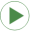IncompressibleFluidNetwork Modelica.Fluid.Examples Multi-way connections of pipes and incompressible medium model

# Used in Components (10)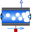EquilibriumDrumBoiler Modelica.Fluid.Examples.DrumBoiler.BaseClasses Simple Evaporator with two states, see Astroem, Bell: Drum-boiler dynamics, Automatica 36, 2000, pp.363-378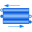BasicHX Modelica.Fluid.Examples.HeatExchanger.BaseClasses Simple heat exchanger model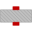WallConstProps Modelica.Fluid.Examples.HeatExchanger.BaseClasses Pipe wall with capacitance, assuming 1D heat conduction and constant material properties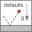System Modelica.Fluid System properties and default values (ambient, flow direction, initialization)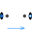PartialTwoPortFlow Modelica.Fluid.Pipes.BaseClasses Base class for distributed flow models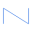PartialStaggeredFlowModel Modelica.Fluid.Pipes.BaseClasses.FlowModels Base class for momentum balances in flow models PartialLumpedVolume Modelica.Fluid.Interfaces Lumped volume with mass and energy balance PartialLumpedFlow Modelica.Fluid.Interfaces Base class for a lumped momentum balance PartialDistributedVolume Modelica.Fluid.Interfaces Base class for distributed volume models PartialDistributedFlow Modelica.Fluid.Interfaces Base class for a distributed momentum balance